﻿ 第四章 运算符，表达式，语句

## 4.1C运算符与分类

int a;

int b = 100;

 运算符 结合律 () [] -> . 从左到右 ! ~ ++ -- +（正号） -（负号） *（指针取值符） (type) sizeof & 从右到左 * / % 从左到右 + - 从左到右 <<   >> 从左到右 < <= >   >= 从左到右 == != 从左到右 & 从左往右 ^ 从左往右 | 从左往右 && 从左往右 || 从左往右 ?: 从右往左 =  +=  -=  *=  /=  %=  &=  ^=  |=  <<=  >>= 从右往左 , 从右往左

下面来逐一的介绍每一个C语言操作符的使用方法。注意：由于某些操作符和后面的C语言知识息息相关，比如位运算操作符，所以目前大家可能对它还比较陌生，但没有关系，等具备了更多的相关知识，再来学习，就问题不大了。

()：扩号运算符

int a = 2;

int b = 1;

int c = 10;

(a+b)*c;

printf(“hello world”);

[]：数组下标运算符

int a = {0};

a = 10;

->：成员选择运算符（左边一般为结构体指针）

.：成员选择运算符（左边一般为结构体变量）

!：逻辑非运算符，将真值变为假值，将假值变为真值。

int a = 10;

if (!(a==0))//if中的表达式的值为true

{

}

~：按位取反运算符

int a = 10;

~a;

++：自增运算符

int a = 10;

a++;

int b = a++;

int c = ++a;

a++;

++a;

int b = a++;//此时相当于：b=a;a = a+1;

int c = ++a;//此时相当于：a=a+1;c=a;

--：自减运算符

int a = 10;

a--;

int b = a--;

int c = --a;

a——a的区别与a++++a类似。

+：正号运算符

-：负号运算符

int a=10;

int b = -a;

*：取值运算符

&：取址运算符

参见第八章指针中会详细介绍。

(type)：强制类型转换运算符

int a = 10;

float f=(float)a;

sizeof：长度运算符

int a = 10;

int length = sizeof(a);

int len = sizeof(int);

*：乘法运算符

int a = 10;

a = a*10;

/：除法运算符

int a = 10;

int b = a/3;

int c = a/5;

%：取余运算符

int a = 10;

int b = a%5;

int c = a%3;

+：加法运算符

int a = 10;

int b = 20;

int c = a + b;

-：减法运算符

int a = 10;

int b = 5;

int c = a – 5;

<<：左移运算符

>>：右移运算符

<：小于运算符

<= ：小于等于运算符

>：大于运算符

>=：大于等于运算符

==：等于运算符

!=：不等于运算符

int a = 10;

int b = 20;

if (a > b)

{

}

if (a >= b)

{

}

if (a < b)

{

}

if (a <=b )

{

}

if (a != b)

{

}

if (a==b)

{

}

在数学里类似的表达式a<b<c，在程序设计语言里不能这样写，而应该写成：

a<b &&b<c

int a = 0;

if (a == 1)

{

}

else

{

}

int a = 0;

if (a = 1)

{

}

else

{

}

1==a

1=a

&：按位与运算符

^：异或运算符

|：按位或运算符

&&：逻辑与运算符

||：逻辑或运算符

int a = 10;

int b = 20;

if (a>b && a>0)

{

}

if (a<b || b > 0)

{

}

&&运算符中，比如：a&&b&&c，假如计算出左边的某个值为假，那么就没有必要计算后面的表达式了。比如，假如a为真，那么继续计算b的值，如果b为真，那么就计算c的值，如果abc都为真，那么整个表达式就为真。而一旦a为假，那么就不用计算b c了，整个表达式就为假。

||运算符中，比如：a || b ||c，假如计算出左边的某个值为真，那么就没有必要计算后面的表达式了。比如假如a为假，那么继续计算b的值，假如b 为假，那么就计算c的值，如果abc都为假，那么整个表达式的值就为假。而一旦a为真，那么就不用计算bc的值了，整个表达式就为真。

?:：三元运算符

2个数最大值：

int a = 10;

int b = 20;

a>     b?a:b;

year%(year%100?400:4)?1:0

=：赋值运算符

int a = 10;

int b = a;

+=

-=

*=

/=

%=

&=

^=

int a = 10;

a += 10;//等价于a = a+10;

a *= 10;// 等价于a = a*10;

a -= 10;// 等价于a = a -10;

a /= 10;// 等价于a = a / 10;

a %= 10;// 等价于a = a %10;

a &= 10;// 等价于a = a&10;

a ^= 10;// 等价于a = a^10;

a |= 10;// 等价于a = a | 10;

## 4.2运算符的优先级与结合律

C语言中，每一种运算符有自己的优先级，还有对应的结合律，每个运算符的优先级和结合律请见上表：表中同一行的运算符优先级相同，并大于下面的运算符，从表中可以看出运算符的优先级，而结合律分为两种：从左到右结合或者从右到左结合，其中大部分是从左到右结合，只有一目运算符，?:运算符，符合运算符如+=，赋值运算符=，以及逗号运算符，是从右往左运算符，剩余的都是从左到右运算符。

5+4*3

1+2+3+4

1+ 8/ 2 *4

3+5*4

(3+5)*4

 口诀 解释 扩建点 （扩建新的地点） 扩（大、中、小括号）建（->符号）点（.符号） 单算易比较 （单独计算容易比较） 单（单目运算符）算（算术运算符）易（移位运算符）比较（比较运算符） 胃饥三等点 （胃很饥饿，等到3点多） 胃（位运算符）饥（逻辑运算符）三（三目运算符）等（赋值运算符，＋＝、－＝等缩写运算符）点（逗点运算符）

## 4.3表达式与求值

C语言中，表达式由运算符、常量及变量构成。每一个表达式，都有对应的一个值。该值与表达式中操作符的优先级和结合律有关。大家已经学习了运算符的概念，那么在本章将进一步学习各种不同的表达式。

a = 0

a + b

a*b

a/b

a%b

a,b,c,d

int main(void)

{

int a,b,c;

a=b=1;

c=a++,b++,++b;

printf("%d,%d,%d\n",a,b,c);

return 0;

}

c=a++,b++,++b

(c=a++),b++,++b

(c=a; a++),b++,++b

c=1, a=2, b= 3

int main()

{

int a=2,b=4,c=6,x,y,z;

z=(y=(x=a+b),(b+c));

printf("y=%d,x=%d,z=%d",y,x,z);

return 0;

}

printf("%d  %d  %d"abc)

func(a, b,c);

a&&b&&c

a||b||c

a>b

a==b

a!=b

a+5*b+(c>>2)

a==NULL

a==true

a==false

a==0

float a;

if (a>-1e-6 && a<1e-6)

{

}

if (a == 0.0)

{

}

fabs(a) == 1e-6

fabs(a) != 1e-6

fabs(a) > 1e-6

fabs(a) < 1e-6

## 4.4语句的三种结构

C语言里，语句是程序的执行单元，程序的功能由程序中的每一条语句实现和完成。程序员写程序，也是通过每一条语句来体现程序的逻辑。

C语言中，每条语句都以分号结束。比如：

C程序的语句，从程序执行流程来看，可以分为顺序结构，语句从上往下顺序执行；分支结构，根据不同的条件判断，执行不同分支部分的语句；循环结构，在满足循环条件下，反复执行一组语句。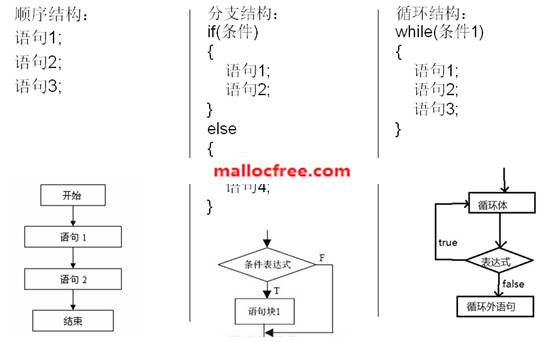## 4.5表达式语句

1，赋值语句：

x=y+z

a=520

2，算术语句：

y+z

3，自增语句，是先取i的值后再加1

i++

4，自增语句，是先把i值增1后再取i的值：

++i

## 4.6函数调用语句

printf(“Hello world\n”);

add(a,b);

## 4.7控制语句

### 4.7.1if语句

if语句是最常见的一种条件判断语句。当对应的条件成立，则执行对应的代码。if语句的格式如下：

if(条件1)

{

语句;

}

if(条件1)

{

语句1;

}

else

{

语句2;

}

if(条件1)

{

语句1;

}

else if (条件2)

{

语句2;

}

else

{

语句3;

}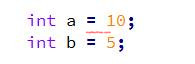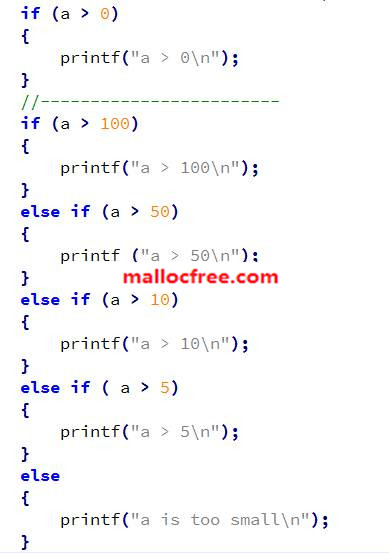### 4.7.2switch语句

switch语句的格式为：

switch(变量)

{

case 变量值1

语句；

Break

case 变量值2

语句；

Break

default:

语句；

}

switch语句的每个case分支，都应该有break语句，这样就不会执行break下面的其它语句了，否则，程序在执行完一个case的所有语句后，会紧接着执行下一个case语句。switch中间哪些类型的变量可以？char,short,int,long可以，但指针和浮点数不可以。Switch语句中当变量的值为下面case中某个值的时候，就会执行该case下的语句，直到遇到break语句。如果所有case的值都不相等，就执行default下面的语句。

char  c;

scanf_s(“%c”,&c,1);//从键盘输入一个字符作为变量c的值。

switch(c)

{

case ‘A’://输入A

语句;

break;

case ‘B’://输入B

语句;

break;

case ‘C’://输入C

语句;

break;

default:

;

}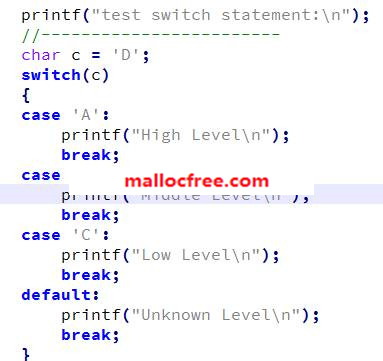### 4.7.3for循环语句

for语句的书写格式如下：

for(表达式1;表达式2;表达式3)

{

语句;

}

for(int i=0;i<10;i++)

{

printf(“%d\n”,i);

}

for语句的三个表达式都可以缺省，如下：

for(;;)

{

}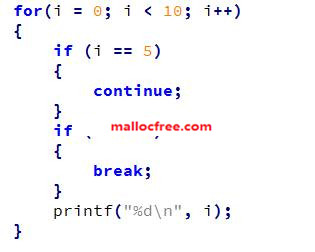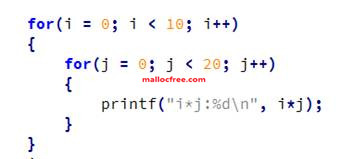### 4.7.4while循环语句

while语句的书写格式如下：

while(条件)

{

语句;

}

while(1)

{

}

while(1)循环因为条件为1，恒为真，所以是一个死循环，需要在循环内部使用break或者return语句跳出循环。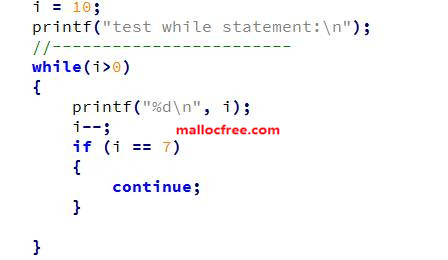### 4.7.5do-while循环语句

do-while语句的书写格式如下：

do

{

语句;

}while(条件);//注意此处需要有分号，否则编译会报错

do-while()循环是先执行语句，再进行条件判断，所以无论条件是否成立，语句都会执行至少一次。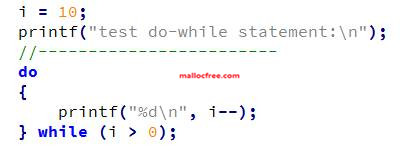i=0;

do

{

printf("%d\n",i);

}while(i>0);

do

{

printf("hello world\n");

}while(0);

1．数组

for (int i = 0; i < n; i++)

{

printf(“%d\n”, a[i]);

}

2．字符串

while (*str !=’\0’)

{

str++;

}

3．链表

while (p)

{

p = p->next;

}

4．栈空/栈满/队空/队满

while(栈非空)

{

出栈；

操作出栈元素

}

5．指针大小比较

while (pStart < pEnd)

{

pStart++;

}

6．数为正

while (size--)

{

}

do

{

}while(size--)

7．反复循环直到条件成熟

while(1)

{

if (something is true)

break;

}

熟练各种循环语句的写法，为实现各种复杂的算法奠定了基础。因此，要在各种循环语句上下功夫，达到熟练书写，灵活运用的程度。

### 4.7.6转向语句：continue/break/goto/return

1break语句

break语句的格式为：

break;

break语句一般用在循环语句来退出循环，或者用在switch语句中，用来退出switch语句的执行。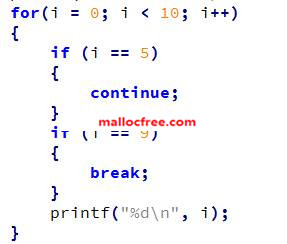2goto语句

C语言中的goto语句是用来控制程序执行从一个地方跳转到另外一个地方执行的。其应用格式为：

LabelA:

语句3;

……

goto LabelA;

l         跳出循环

for(int i=0;i<10;i++)

{

for(int j=0;j<10;j++)

{

for(int k=0;k<10;k++)

{

if(k==5)

{

goto out;

}

}

}

}

out:

l         出错处理

char *p1=(char *)malloc(128);

if(p1==NULL)

goto exit;

char *p2=(char *)malloc(16);

if(p2==NULL)

goto exit;

exit:

if(p1)

free(p1);

if(p2)

free(p2);

3continue语句

while循环为例子，语句continue在循环中的使用格式为：

while(条件1)

{

if(条件2)

{

continue;//当条件2位真，那么循环后面的其它语句就不会再执行

//而重新判断条件1是否为真

}

其它语句;

}

int i=10;

while(i>0)

{

i--;

if(i==7)

{

//i等于7的时候，下面的printf函数语句会被忽略

//所以不会打印出7这个数字

continue;

}

printf(“%d\n”,i);

}

4return语句

return 表示从被调函数返回到主调函数继续执行，返回时可附带一个返回值，由return后面的参数指定，return返回的值类型由函数声明中的返回类型确定。比如：

1）在返回类型是char的函数中，return后应该是char类型的值；

2）在返回类型是int的函数中，如果是要停止函数的调用，最好应该为0；其他的按照你的目的而定，只要是int 类型就行了；

3）在返回类型是结构类型的函数中，return后应该是结构的一个实例对象；

4return后面也可以跟表达式，比如函数调用等；

4）如果函数无返回类型，而是void，则无需要返回任何类型的值。

return语句的使用格式：

{

类型 value;

……

……

return value;

}

int func(参数列表)

{

int result = 0;

……

return result;

}

## 4.8空语句

while(*str1++==*str2++ &&

*str1&&
*str2)

## 4.9复合语句

{

x=y+z

a=b+c

printf(%d%d”，xa)

}

上面的代码就是一条复合语句。复合语句内的各条语句都必须以分号“；”结尾；但在括号“}”外不能加分号。

## 4.10代码风格

1．  注释

注释有两种方式，它们分别是：

方式1

/*

*  this is a comment

*  the following code will do something

*  email:xxxx@126.com

*/

/*  this is a comment  */

//  this is a comment

1./*

2. * xxxx

3. /*

4.  * yyy

5.  */

6. * zzz

7.*/

17行的注释中嵌套了35行的注释。那么注释将在第5行终止。第67行将失去注释作用。

2．  断行

为了便于阅读，一行代码不要太长。在Solaris内核代码中，要求一行不应超过80个字符。断行时应该选择将操作符留在最右边。新起一行应该在原来行缩进的基础上空4个字符。    例如：

if(pEnumProcesses == NULL ||

pGetModuleFileName == NULL ||

pEnumProcessModules == NULL)

{

return;

}

3．  每行只写一条语句

int a, b, t;

t = a; a = b; b = t;

int a;

int b;

int t;

t = a;

a = b;

b = t;

这样写很方便注释与调试。

4．  分行定义变量

参加条例3的定义

5．  使用空行分隔代码块

将完成同一逻辑的代码放在一个段中。适当地添加空行则是一个非常有效的代码整理方式——有点像文章中的分段，一段意思也许需要若干个句子才能表达清楚，在表达下一段意思之前，应当另起一段。

6．  使用空格降低代码密度

运算符与操作数之间以空格间隔；关键字与非关键字之间空格间隔。但单目运算符与它的操作数之间应紧密相接，不需要空格。括号（包括小括号、中括号与大括号）的内侧应该紧靠操作数或其他运算符，不需要添加额外的空格。不要在函数名和其后的括号之间添加空格。

需要空格的位置：

1）  forifwhileswitch等关键字与之后的左括号’(‘之间，如：

while (b)

{

}

2）  左花括号’{‘之前，如：

if (b) {

}

3）双目运算符两侧，例如：

p == NULL;

a += b;

4）逗号’,’与分号’;’之后，如：

for (i = 0; i < 10; i++)

{

}

printf(“%d, %c\n”, a, ch);

不要空格的位置：

1）  函数名与之后的左括号’(‘，包括带参数的宏与之后的左括号’(‘，如：

max(a, b);

2）分号’;’与冒号’:’之前，如：

a = b;

switch (i)

{

case 1:

break;

defaut:

break;

}

3）左括号’(‘右边，右括号’)’左边，如：

if (p == NULL)

4）单目运算符，如：

i++ !b

1）  关键字与其他字符之间，如：

int length = sizeof (b);

其中的intsizeof都是关键字。

2）  括号（大，中，小）内侧：

int a;

if (a >= b)

{

return (a);

}

7’.’’->’运算符不需要空格，如：

if (b->left)

{

}

list.val = 0;

需要空行的位置：

1）函数的定义之前、函数的定义之后；

2）一组联系紧密的代码段之前和之后。

/* other code above */

void swap(int a, int b)

{

int t;

t = a;

a = b;

b = t;

return;

}

/* other code below */

7．  return

return (a);

return (NULL);

return (a + b);

return;

8．  缩进

是通过在每一行的代码左端空出一部分长度（一般为一个TAB键），更加清晰地从外观上体现出程序的层次结构。当遇到有关命名空间、类、结构、函数、以及枚举等等复杂程序结构的定义的时候，我们通常需要将它的内容缩进一层。

一般函数体、过程体、循环体(for,while,do)、条件判断体(if)和条件选择(switch,case,select)需要向内缩进一格，同层次的代码在同层次的缩进层上。

if (condition)

{

//缩进

printf(“hello world”);

}

else if (condition)

{

//缩进

}

else

{

//缩进

}

for (int i = 0; i < n; i++)

{

//缩进

}

while (condition)

{

//缩进

}

do

{

//缩进

} while(conditon)

switch(var)

{

case a:

//缩进

break;

case b:

//缩进

break;

default:

//缩进

break;

}

int function(int a, int b)

{

//缩进

}

class MyClass

{

//缩进

}

struct MyStruct

{

//缩进

};

9．  大括号

if (condition) {

}

else if (condition) {

}

else {

}

for (int i = 0; i < n; i++) {

}

while (condition)

{

}

do

{

} while(conditon)

switch(var)

{

case a:

break;

case b:

break;

default:

break;

}

int function(int a, int b)

{

}

class MyClass

{

}

struct MyStruct

{

};

10．              NULL0FALSE, 100等常量与变量的比较写法

if (NULL  ==  p)

if (p == NULL)

if (i == 0)

if 0 == i

if  (b) if(b==TRUE)

if (!b)  if(b==FALSE)

float f;

if (f == 0)

#define DELTA 0.00001

if (f >= -DELTA && f <= DELTA)

int a = 10;

if (a==0)

{

}

else

{

}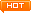看文字不过瘾？点击我，进入周哥教IT视频教学